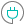#CHIINV (PQL - xl)

This function calculates the inverse of the one-tailed probability of the chi-squared distribution

#### Syntax

CHIINV(probability, deg freedom)

##### Function Arguments
 Name Description Type Optional probability Probability of the chi-squared distribution Number deg freedom Number of degrees of freedom; if not an integer, the number is truncated Number